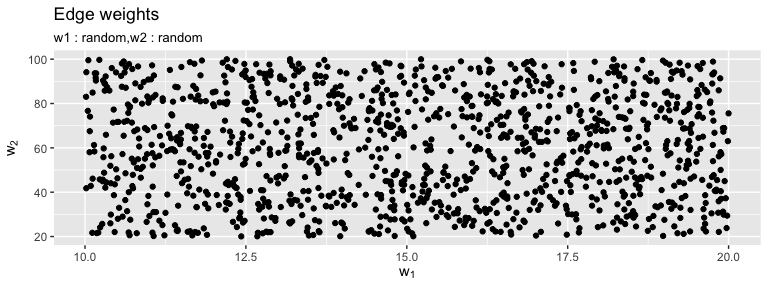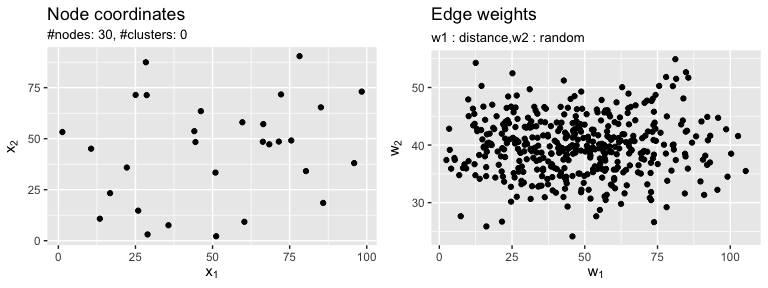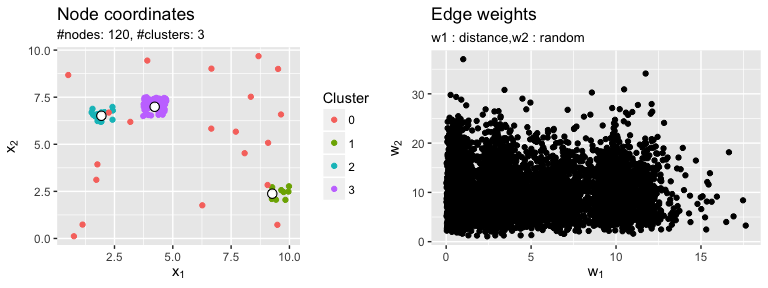# Benchmarking optimization algorithms

Benchmarking algorithms for (combinatorial) optimization problems is usually carried out by running the set of algorithms on a set of test problems. Often, due to lack of real-world data, the test set consists of artificially generated benchmark problems. Artificial problems allow for 1) the generation of arbitrary many instances in short time and 2) the generation of problems of different hardness levels by implementing theoretical knowledge. E.g., for the mcMST problem it is known, that if all edges of a complete graph are pairwise incomparable, all spanning trees are nondominated. Contrary, if there are many dominated edges, the number of nondominated trees tends to be smaller. This way we can generate problems with different sizes of the Pareto-set.

The mcMST package implements a modular and flexible approach to the generation of multi-objective graph problems. The first step is to generate a bare graph skeleton with no clusters, node coordinates, edges or edge weights associated. All these components are stacked on top of the skeleton step by step allowing for great flexibility.

## Examples

We start of with a bi-objective problem with n = 50 nodes where both weights are drawn at random from a uniform distribution R(10, 20) and R(20, 100) respectively.

library(mcMST)

g = mcGP(lower = 0, upper = 1)
g = addWeights(g, method = "random", weight.fun = runif, min = 10, max = 20, n = 50)
g = addWeights(g, method = "random", weight.fun = runif, min = 20, max = 100)
plot(g)\$pl.weightsThe next example is a little bit more complex. Here, a bi-objective graph with n = 30 nodes is generated with node coordinates in the euclidean plane [0, 100] x [0, 100]. The first weight function is the euclidean distance between the points in the plane. The second weight is normally distributed with mean 40 and standard deviation 5.

g = mcGP(lower = 0, upper = 100)
g = addCoordinates(g, n = 30, generator = coordUniform)
g = addWeights(g, method = "euclidean")
g = addWeights(g, method = "random", weight.fun = rnorm, mean = 40, sd = 5)
print(g)
#> MULTI-OBJECTIVE GRAPH PROBLEM
#> Number of nodes: 30
#> Weights per edge: 2 (distance,random)
library(gridExtra)
do.call(grid.arrange, c(plot(g), nrow = 1))Finally we generate a bi-objective clustered instance. Here, we first place 3 cluster centers by means of a Latin hypercube sample in [0, 10] x [0, 10]. In the next step we place 120 nodes in total: 10 around the first, 20 around the second and 70 around the third cluster center. Additionally we sample random node coordinates in [0, 10] x [0, 10]. The first weight is the Manhatten block distance between the node coordinates. The second weight is drawn from a Cauchy distribution with 10 degrees of freedom.

g = mcGP(lower = 0, upper = 10)
g = addCenters(g, n.centers = 3, generator = coordLHS)
g = addCoordinates(g, n = c(10, 20, 70), by.centers = TRUE, generator = coordUniform,
lower = c(0, 0), upper = c(1, 1))
g = addCoordinates(g, n = 20, generator = coordUniform)
g = addWeights(g, method = "manhattan")
g = addWeights(g, method = "random", weight.fun = rchisq, df = 10)
do.call(grid.arrange, c(plot(g), nrow = 1))Graph problems with more than two objectives are possible as well. However, the plot function does not support these at the moment.